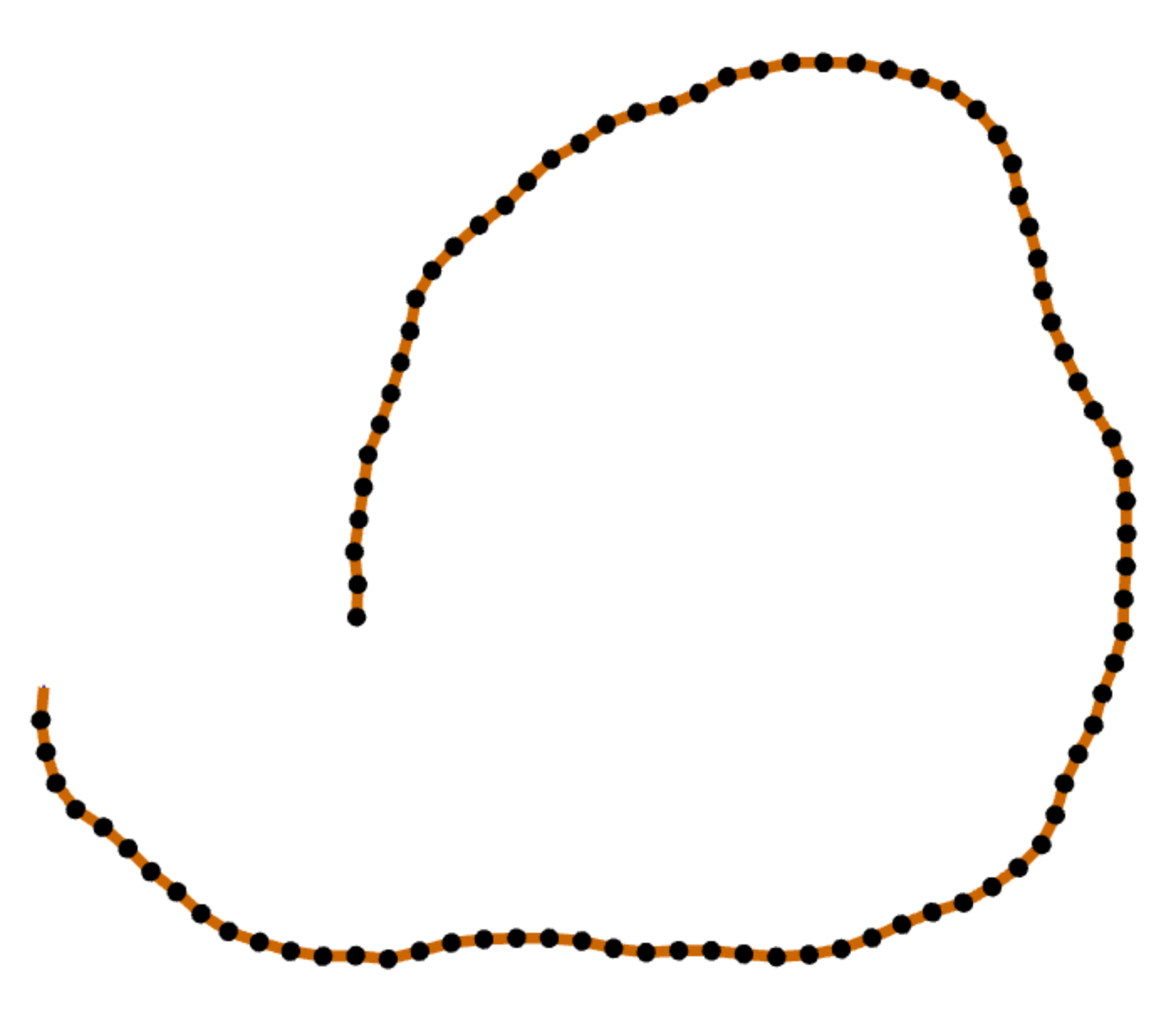# teaching machines

## Random Splats

I keep a thinklist of things I’d like to think about. This list comes in handy during meetings. Sometimes I even look forward to meetings, because that means I’ll have some time to think.

Recently I added to this list the notion of generating random splats. My first thinking was to generate a circular polyline and then use smooth noise to perturb its vertices. To keep the perturbations aesthetically pleasing, however, I would need to increase the density of the samples along the splat’s perimeter. Uniform sampling would produce noticeably jagged edges when the vertices were pushed around.

My second thinking was to try a random walk. I would maintain a constant length for all segments of the splat’s perimeter. I wrote a little program for that in Madeup. The core idea is to take a step and then turn a random amount. The turning angle is more likely to be a right turn then a left turn—meaning that we will generally sweep out a clockwise turn. But when do we stop our walk? Here I stopped when we netted 360 degrees in our turn:

seed = 102

angle = 0
moveto 0, 0
while angle < 360
delta = random -10, 20
yaw delta
move 10
angle = angle + delta
end

extrude 0, 0, 1, 50But this isn’t a closed path, which makes for a sorry splat:My third thinking came on a train between San Francisco and Palo Alto. I considered how I could ensure that the random walk stopped in the same place it started. I wondered if I could amortize the gap between the start and end points across all the intermediate points. I had to make some tweaks to Madeup to support this, but the result is as I hoped:



seed = 120
.rgb = {0.8, 0.3, 0.6}

-- Go for a random walk.
angle = 0
moveto 0, 0
while angle < 360
delta = random -5, 10
yaw delta
move 10
angle = angle + delta
end

-- Convert the path into an
-- array of vertices.
vertices = path

-- How much space is between
-- the start and end?
gap = vertices[-1] - vertices

-- Let's divide that gap by
-- the number of vertices we
-- have...
shift = -gap / size vertices

-- Shift each vertex over by
-- a fraction of the gap.
moveto 0, 0
for i to size vertices
vertex = vertices[i]
translate shift, shift, 0
moveto vertex, vertex, vertex
end

extrude 0, 0, 1, 50

var mupDiv = jQuery('#mup_disconnected');
mupDiv.closest('pre').replaceWith(mupDiv);
document.getElementById('mup_form_disconnected').submit();

seed = 120
.rgb = {0.8, 0.3, 0.6}

-- Go for a random walk.
angle = 0
moveto 0, 0
while angle < 360
delta = random -5, 10
yaw delta
move 10
angle = angle + delta
end

-- Convert the path into an
-- array of vertices.
vertices = path

-- How much space is between
-- the start and end?
gap = vertices[-1] - vertices

-- Let's divide that gap by
-- the number of vertices we
-- have...
shift = -gap / size vertices

-- Shift each vertex over by
-- a fraction of the gap.
moveto 0, 0
for i to size vertices
vertex = vertices[i]
translate shift, shift, 0
moveto vertex, vertex, vertex
end

extrude 0, 0, 1, 50



Thank you, CalTrain. Next on my thinklist is adding lobes to my random splats.